# What are the C programming concepts used as Data Structures

• Difficulty Level : Easy
• Last Updated : 13 Dec, 2021

## Data Types

Data-type in simple terms gives us information about the type of data.
Example, integer, character, etc.
Data-types in C language are declarations for the variables. Data-types are classified as:

#### Primitive or Built-in data types

Some of the examples of primitive data types are as follows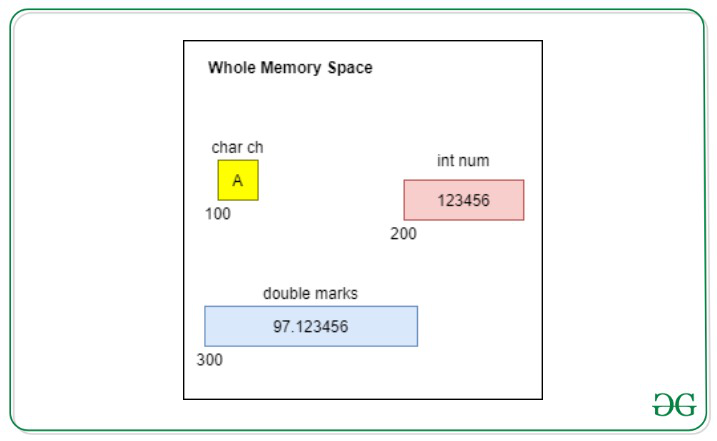Variable named ch refers to the memory address 100, has occupied 1 byte of memory, which holds the value of char datatype ‘A’.

num is of integer type referring to the memory address 200, has occupied 4 bytes of memory, and holds value 123456.

marks is of double type, referring to the memory location 300, has occupied 8 bytes of memory, and holds value 97.123456.

Note: These addresses(100, 200 & 300) are just for understanding purpose, actual addresses are large hexadecimal numbers.

## Arrays

An Array is a variable that can store multiple values of the same datatype. Array is a contiguous memory segment.

Example:

If you want to store 100 integers, you can use one array instead of using 100 different integer variables.

Syntax for declaration of array:

`data_type   array_name[array_size];`

Amount of space allocated = sizeof(data_type) * array_size

Example:
Declare an array that can hold values of 4 integers

`int arr;`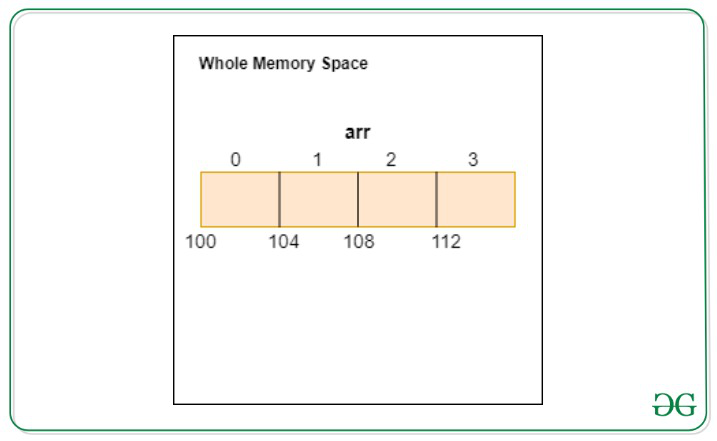In this case, sizeof(int) = 4. Therefore, 4*4 = 16 bytes of memory is reserved for array.

Array declaration & Initialization Example:

`int arr = {10, 20, 30, 40, 50};`

Array elements can be accessed using indices, which range from 0 to (array_size-1).

Below is the sample code for the usage of arrays in C:

## C

 `// C implementation to demonstrate``// the usage of arrays` `#include ` `int` `main()``{``    ``// Array Indexs-0   1   2   3   4``    ``int` `arr = { 10, 20, 30, 40, 50 };` `    ``int` `size = ``sizeof``(arr);` `    ``printf``(``"Size of array is %d\n"``, size);` `    ``printf``(``"Data at index 2 is %d\n"``, arr);``}`

Output

```Size of array is 20
Data at index 2 is 30```

## Structures

We have used primitive data types to store data. But what if the data we want to store is more complex?

Let’s consider an example, we want to store information of the students in a class in a single variable. So, a student has :

• Roll Number
• Name

Here, Roll number is of integer type and Name is string(array of characters) type.

Here, is the solution: structures

• Structure is a collection of variables(can be of different data types), under a single name.
• It is also a contiguous memory segment like array, but it allows data members of different data types.

Syntax to define structures:

```struct structure_name
{
datatype member1_name;
datatype member2_name;
..
datatype membern_name;
};```

Example:

```struct student
{
int roll_number;
char name;
};```

Now we have newly defined data type, struct student. We can create it’s variables.

Syntax for variable declaration:

`struct structure_name variable_name;`

Example:

```struct  student  ram;

// Members of structures can
// be accessed using "." operator
stu.roll_number = 64;
stu.name = “Saurabh”;```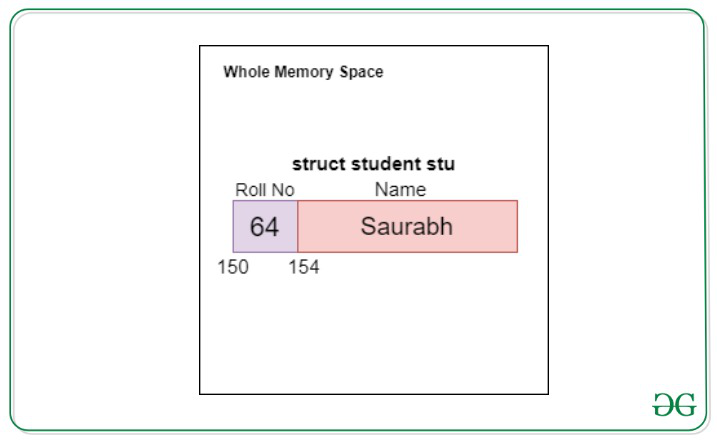No memory is allocated when we define a structure.

Size of structure is equal to total amount of space consumed by each data member.

Example:

In case of student structure, it is 4 + 20 = 24 bytes

Below is the illustration of structures with the help of code:

## C

 `// C implementation to demonstrate``// the usage of structures` `#include ``#include ` `// Structure Definition``struct` `student {``    ``// data members``    ``int` `roll_no; ``// 4 bytes``    ``char` `name; ``// 20 bytes``};` `int` `main()``{``    ``// Structure variable Declaration``    ``struct` `student stu;` `    ``stu.roll_no = 64;``    ``strcpy``(stu.name, ``"Saurabh"``);` `    ``printf``(``"Structure Data\n"``);``    ``printf``(``"Roll No: %d\n"``, stu.roll_no);``    ``printf``(``"Name: %s\n"``, stu.name);` `    ``int` `size = ``sizeof``(stu);` `    ``printf``(``"Size of Structure student"``);``    ``printf``(``"is %d"``, size);``}`

Output

```Structure Data
Roll No: 64
Name: Saurabh
Size of Structure studentis 24```

## Pointers

• Pointers are the special type of variables that stores the address, rather than the value of the variable.
• They are used for indirect access of variables.
• If var is the name of variable, then &var gives the address of var.

Remembered the ampersand(&) symbol used in scanf function

`scanf(“%d”, &var);`

This is because we assign the values scanned to the memory location of var.

We are not interested in addresses, but the values stored at that address.

Syntax for declaration of pointer:

`data_type* pointer_name; // (* = asterisk)          `

Example:

`int* ptr;`

Pointer may point to any datatype
It can hold address of any variable of the datatype it is pointing to.
An uninitialized pointer variable has NULL value.

Example:

```int* ptr;
int num = 5;
ptr = &num;```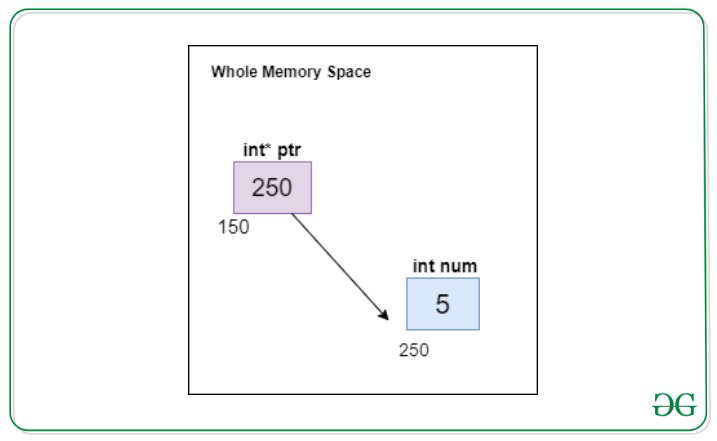To get the value at the address, the pointer is pointing to, we use asterisk(*) operator.

So, in the above example, the ptr is holding address 250 and the value at address is 5.

Hence, *ptr is equal to 5.

Below is the illustration of the pointers with the help of code:

## C

 `// C implementation to demonstrate``// pointers in C` `#include ` `int` `main()``{``    ``int``* ptr;``    ``int` `num = 5;` `    ``ptr = #` `    ``// This gives address of num``    ``printf``(``"Value at ptr is %p\n"``, ptr);` `    ``// This gives value at num``    ``printf``(``"Value at *ptr is %d\n"``, *ptr);``}`

Output

```Value at ptr is 0x7ffdff4dca9c
Value at *ptr is 5```

Size of Pointer variable is always constant in a system, irrespective of the datatype it is pointing to and it is usually 8 bytes.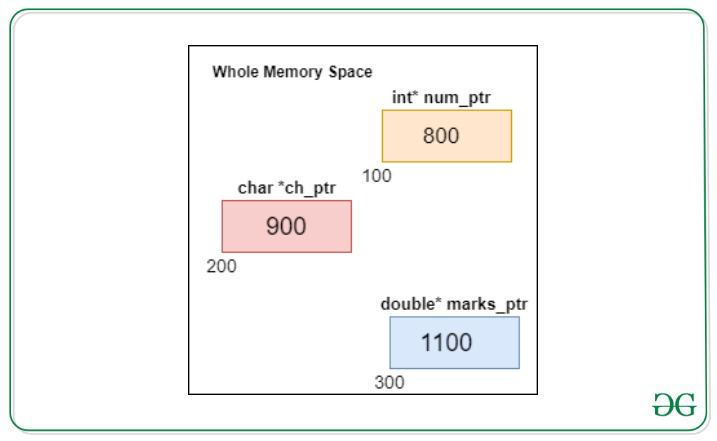### Pointer to the Structure

• Pointer to the structure can be declared as normal variable.

Example:

`struct student *p;`

Here,  p is pointer and *p is the structure

Hence, to access the data members, we have to use

```(*p).roll_no
(*p).name```

C provides a special operator for accessing the data members via pointer i.e. -> arrow operator.

Note: (*p).x equivalent to p->x

Below is the illustration of the pointers to the structure:

## C

 `// C implementation to illustrate``// the code of the structures` `#include ``#include ` `// Structure Definition``struct` `student {``    ``int` `roll_no;``    ``char` `name;``};` `int` `main()``{``    ``struct` `student* p;` `    ``p = (``struct` `student*)``        ``malloc``(``sizeof``(``struct` `student));` `    ``// Arrow operator``    ``p->roll_no = 99;` `    ``printf``(``"The value at roll"``);``    ``printf``(``"number is %d"``, p->roll_no);` `    ``return` `0;``}`

Output

`The value at rollnumber is 99`

## Functions

• A function is a block of code that performs a specific task.
• A function may have an input, performs so tasks, then may provide some output.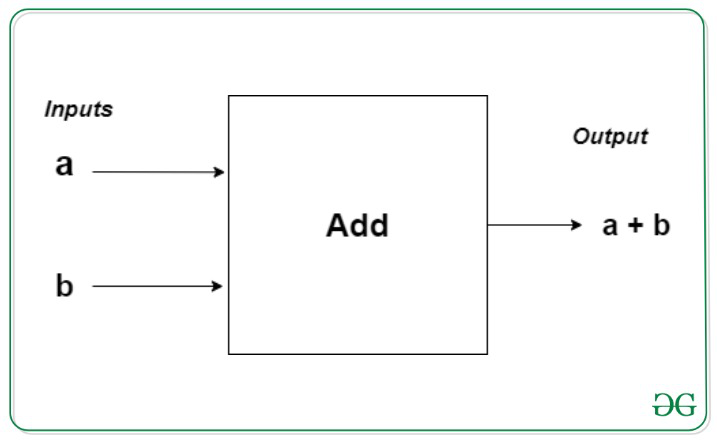In the above example, we are giving inputs as 2 numbers to a function. It is performing function of addition. Then, it is return the sum of the two inputted numbers.

• Inputs are called are parameters of the function
• Output is called a return value

Functions can be classified into two categories:

### Built-in or predefined functions

These are defined in the standard library of C language. We don’t have to define these functions, only thing needed is to call these functions. We just need to know the proper syntax and we can readily use these functions.

Example:

printf(), scanf(), main(), etc are the predefined function.

### User-defined functions

• These are the function, defined by the programmer to execute some task in the program.
• Dividing a complex problem into smaller chunks makes our program easy to understand.

To use the user-defined function, we have to perform two steps

1. Defining function
2. Calling function

Syntax of function definition:

```return_type  function_name(<parameters_list>)
{
return return_value;
}```

Note:

1. A function can have 0 or more parameters.
2. A function can have 0 or 1 return value.
3. Function that doesn’t return anything has return type void.

Below is the illustration of the functions in C:

## C

 `// C implementation to``// illustrate functions in C` `#include ` `// program to demonstrate functions``// function definition``// function to print something` `void` `print()``{``    ``printf``(``"GeeksforGeeks\n"``);``}` `// Function to add two numbers``int` `add(``int` `a, ``int` `b)``{``    ``int` `sum;``    ``sum = a + b;``    ``return` `sum;``}` `// Main Function``int` `main()``{``    ``int` `res;` `    ``// Function call``    ``print();` `    ``res = add(5, 7);` `    ``printf``(``"Sum is %d"``, res);``}`

Output

```GeeksforGeeks
Sum is 12```

Note: The type passed in the function call should be compatible with the received by the function body as a parameter. Else, it will cause compilation error.

### Function Classification based on type of call:

#### Function call by passing value

• When we call function by passing value(as in above program), values of original variables are unaffected.
• Instead of sending original variable, it sends copy of a variable.

Below is the illustration of the function call by passing value in C:

## C

 `// C implementation for the``// function call by passing value` `#include ` `// Function pass by value``void` `increase_by_one(``int` `x)``{``    ``x++;``}` `int` `main()``{``    ``int` `num = 5;` `    ``printf``(``"Value before function"``);``    ``printf``(``" call %d\n"``, num);` `    ``increase_by_one(num);` `    ``printf``(``"Value after function"``);``    ``printf``(``" call %d\n"``, num);``}`

Output

```Value before function call 5
Value after function call 5```

In this program, we have passed variable a to the function, which was holding value 5. Then, we incremented the value of variable received by function i.e. x by 1. So, value of x now is 6. But, this change is limited to function scope only. The value of a in main will still be 5.

#### Function call by passing address

• If we want to change value of the variable passed, we have to pass the variable by address.
• This method uses the same variable instead of creating its new copy.

Below is the code of the function call by passing address:

## C

 `// C implementation to demonstrate``// the usage of function call by``// passing reference` `#include ` `// function to demonstrate``// call by value``void` `increase_by_one(``int``* x)``{``    ``(*x)++;``}` `int` `main()``{``    ``int` `num = 5;` `    ``printf``(``"Value before function"``);``    ``printf``(``" call %d\n"``, num);` `    ``increase_by_one(&num);` `    ``printf``(``"Value after function"``);``    ``printf``(``" call %d\n"``, num);``}`

Output

```Value before function call 5
Value after function call 6```

As we are passing address of variable, we have to receive it as pointer variable.

## C

 `// C implementation to demonstrate``// the example of the passing as``// parameter in the function` `#include ` `// Function to print the array``void` `print_array(``int` `arr[], ``int` `n)``{``    ``// N is size of array``    ``int` `i;` `    ``for` `(i = 0; i < n; i++)``        ``printf``(``"%d "``, arr[i]);``}` `int` `main()``{``    ``int` `arr = { 10, 20, 30, 40, 50 };` `    ``// Function Call``    ``print_array(arr, 5);``}`

Output

`10 20 30 40 50 `

## Type Casting

Type casting is basically conversion of one datatype into another.

Syntax of type casting:

```var2 = (datatype2) var1
where,
var1 is of datatype1 & var2 is of datatype2```

Example:

If you want to convert value of an integer variable into float variable

`float x = (float)7/5;`

To learn more about typecasting, refer Type Conversion in C

## Dynamic Memory Allocation

As you know, an array is a collection of a fixed number of values. Once the size of an array is declared, you cannot change it.

Sometimes the size of an array you declare may be insufficient. To solve this issue, you can allocate memory dynamically at runtime. This is known as dynamic memory allocation.

Predefined functions used in dynamic memory allocation:

### 1. malloc()

• malloc stands for memory allocation.
• The malloc() function reserves a block of memory of the specified number of bytes and void* which can be casted into pointers of any form.

Syntax of malloc():

`pointer_name = (cast_datatype*)malloc(size);`

### 2. free()

• Dynamically allocated memory using malloc() doesn’t get freed on its own. You must explicitly use free() to release this space.

`free(pointer_name);`

Note: These functions are declared in header file stdlib.h. To use these functions, you must first include this header.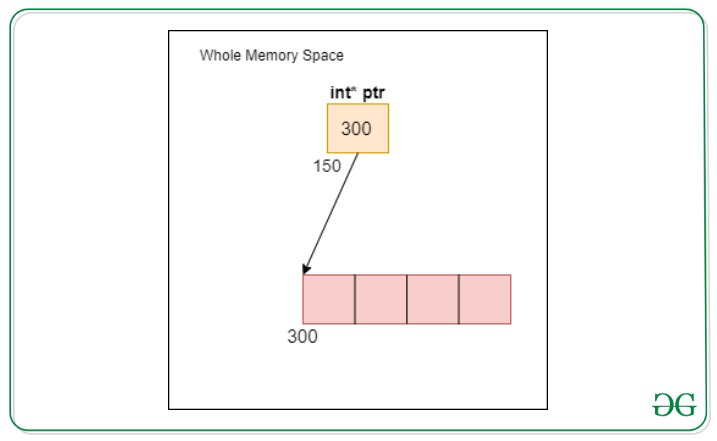Below is the illustration of the Dynamic Memory Allocation in C:

## C

 `// C implementation to demonstrate``// the code the Dynamic Memory``// Allocation` `#include ``#include ` `int` `main()``{``    ``int``* ptr;``    ``int` `n = 5, i;` `    ``ptr = (``int``*)``malloc``(n * ``sizeof``(``int``));` `    ``for` `(i = 0; i < n; i++)``        ``ptr[i] = i;` `    ``printf``(``"\nArray Elements are\n"``);` `    ``for` `(i = 0; i < n; i++)``        ``printf``(``"%d "``, ptr[i]);` `    ``free``(ptr);``}`

Output

```Array Elements are
0 1 2 3 4 ```

To learn more about Dynamic Memory Allocation, refer Dynamic Memory Allocation in C

My Personal Notes arrow_drop_up